Adjustment on the Type I Error Rate for a Clinical Trial Monitoring for both Intermediate and Primary Endpoints | OMICS International
Journal of Biometrics & Biostatistics

Like us on:

# Adjustment on the Type I Error Rate for a Clinical Trial Monitoring for both Intermediate and Primary Endpoints

Susan Halabi*

Department of Biostatistics and Bioinformatics, Duke University Medical Center, USA

*Corresponding Author:
Susan Halabi, Ph.D.
Department of Biostatistics and Bioinformatics
Duke University Medical Center
Durham, NC 27710, USA
E-mail: [email protected]

Received date: April 10, 2012; Accepted date: May 18, 2012; Published date: May 19, 2012

Citation: Halabi S (2012) Adjustment on the Type I Error Rate for a Clinical Trial Monitoring for both Intermediate and Primary Endpoints. J Biomet Biostat S7:015. doi:10.4172/2155-6180.S7-015

Copyright: © 2012 Halabi S. This is an open-access article distributed under the terms of the Creative Commons Attribution License, which permits unrestricted use, distribution, and reproduction in any medium, provided the original author and source are credited.

Visit for more related articles at Journal of Biometrics & Biostatistics

#### Abstract

In many clinical trials, a single endpoint is used to answer the primary question and forms the basis for monitoring the experimental therapy. Many trials are lengthy in duration and investigators are interested in using an intermediate endpoint for an accelerated approval, but will rely on the primary endpoint (such as, overall survival) for the full approval of the drug by the Food and Drug Adminstration. We have designed a clinical trial where both intermediate (progression free survival, (PFS)) and primary endpoints (overall survival, (OS)) are used for monitoring the trial so the overall type I error rate is preserved at the pre-specified alpha level of 0.05. A two-stage procedure is used. In the first stage, the

Bonferroni correction was used where the global type I error rate was allocated to each of the endpoints. In the next stage, the O'Brien-Fleming approach was used to design the boundary for the interim and final analysis for each endpoint. Data were generated assuming several parametric copulas with exponential marginals. Different degrees of dependence, as measured by Kendall's τ , between OS and PFS were assumed: 0 (independence), 0.1, 0.3, 0.5 and 0.7. The results of the simulations were robust regardless of the copula that were assumed. We controlled for the global type I error and marginal type I rates for both of the endpoints under the null hypothesis. In addition, the global power and individual power for each endpoint were attained at the desired level under the alternative hypotheses. This approach is applied to an example in a prostate cancer trial.

#### Keywords

Copula; Censoring; Time-to-event; Primary endpoint; Sequential methods, O'Brien-Fleming boundaries, Two-step procedure

#### Introduction

In many clinical trials a single endpoint is used to answer the primary question and forms the basis for monitoring the experimental drug. Many clinical trials are lengthy in duration and there is widespread interest among clinical investigators and pharmaceutical firms in employing surrogate or intermediate endpoints to assist in making decisions about the efficacy of certain drugs. Thus, more than one primary endpoint may be employed in the design and in the monitoring in the clinical trial. For a drug or a device to be considered efficacious, it must demonstrate tangible clinical benefit, generally defined as an improvement in survival or improvement in symptoms . The Food and Drug Adminstration (FDA) established accelerated approval for oncology products by the Oncology Drug Approval Committee (ODAC) if the product is "reasonably likely to predict clinical benefit or an evidence of an effect on a clinical benefit other than survival'' . As a result, many investigators and pharmaceutical firms are interested in using an intermediate endpoint for an accelerated approval, but will rely on the primary endpoint for the full approval of the drug by the FDA.

In most randomized clinical trials, interim monitoring of data is a common practice if not a requirement. Group sequential designs developed by Pocock  and O'Brien-Fleming  have been widely applied. In addition, the more flexible alpha spending approaches proposed by Lan and DeMets  have been widely implemented. Other designs developed by Pampallona and Tsiatis  and Lakatos , who implemented the group sequential design for survival endpoints, are employed. Whitehead  proposed a straight line approach for comparing survival curves, while Jennison and Turnbull  support the use of repeated confidence intervals for monitoring a trial.

Most of the authors of the literature cited above apply sequential monitoring designs for one endpoint. Jennison and Turnbull described a method for monitoring two endpoints, namely efficacy and safety endpoints . On the other hand, Cook and Farewell proposed an asymmetric procedure to control for the type I error rate for one efficacy and one toxicity response outcome . Todd proposed an adaptive method for monitoring bivariate endpoint that can be extended to the multivariate case .

We sought to design the SPARC trial, a phase III trial in men with castrate resistant prostate cancer (CRPC) who failed first line chemotherapy where both intermediate (progression-free survival, PFS) and primary (overall survival, OS) are used for monitoring the trial. The sponsor believes that there is an unmet need and would like to use the PFS endpoint for accelerated approval, but OS, which requires more follow-up, will be used for full approval of the drug by the FDA. In this study, PFS is defined as time from randomization to time of disease progression (either bone, tumor, clinical or pain) or death. OS is defined as interval between time of randomization to time of death from any cause. Both of these endpoints are time-to-event and there is some degree of dependence between them.

The main questions that we are interested in answering are: 1) how to allocate the type I error rate between these time-to-event endpoints when there is a dependence, 2)what is the impact of univariate monitoring of each endpoint on the global type I error rate and marginal type I error rates for each of the two endpoints, and the proportion of not terminating the trial early and 3)what is the impact of univariate monitoring of each endpoint on the global power and univariate power for each of the two endpoints, and the proportion of terminating the trial early.

In this article, we consider an approach that will allow statisticians to design a trial for monitoring two co-primary time-to-event endpoints when there is a dependence structure. We investigate the global and marginal type I error rates and the power empirically using extensive simulations. Furthermore, we study the operating characteristics under several dependence structures by using parametric copulas. Finally, we investigate the operating characteristics of the design using different allocation of the type I error rate.

#### Methods

A simulation framework that does not incorporate dependency between OS and PFS may be unrealistic. To address this issue, we will employ copulas to generate OS and PFS under various dependence structures. From a statistical perspective, a copula is a bivariate distribution function with uniform marginals. Suppose that (U,V) is a random pair with uniform marginals (i.e, P[U ≤ u] =u for all u ∈ [0,1] and P[V ≤ v]=v for all v ∈ [0,1]). Then the copula, say C, associated with (U,V) is defined as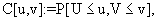(1)

for all (U,V) ∈ [0,1]2. Given a pair of continuous marginal distribution functions, say F1 and F2, the function (U,V) is distributed according to C if and only if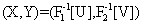is distributed according to H,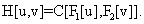(2)

Due to a result by Sklar , for any random pair (X,Y) with marginals F1 and F2, there exists a copula C. Furthermore, the representation is unique if the marginals are continuous.

Suppose that (U,V) is a random pair with uniform marginals generated by copula C. Also, suppose that f and f+ are decreasing and increasing functions from [0,1] into [0,1]. Then U and V are independent if and only if C[u,v]=I[u,v]=uv, U=f[V] almost surely if and only if C[u,v]=L[u,v]=max[u+v−1,0] and U=f+[V] almost surely if and only if C[u,v]=U[u,v]=min[u,v], for all (U,V) ∈ [0,1]2. Furthermore, for any copula L[u,v]≤C[u,v]≤U[u,v] for all (U,V) ∈ [0,1]2. The copulas L and U are called the lower and upper Frechet-Hoeffding bounds.

There exists a rich family of copulas where the dependence structure is parameterized by a single parameter. We will consider three examples. The normal copula is defined as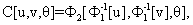(3)

where θ ∈ [−1,1]. Here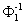denotes the quantile function for a univariate standard normal distribution and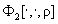denotes the distribution function for a standard bivariate normal distribution with correlation parameter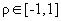. Frank's copula is given as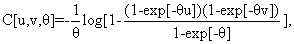(4)

where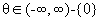. Finally, Gumbel's copulas is given by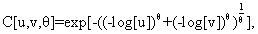(5)

where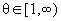.

For the normal copula, C↑(↓)I as θ↑(↓)0 , C↓L as θ↓−1 and C↑U as θ↑1. We are using the up- and down-arrows to denote monotone increasing and decreasing convergence. For Frank's copula, C↑(↓)I if θ↑(↓)0 , C↓L if θ↓−∞ and C↑U if θ↑∞. For Gumbel's copula, C↓I if θ↓1 and C↑U if θ↑∞. Note that unlike the normal and Frank's copula, Gumbel's copula does not admit negative dependence structures.

The dependence parameter in the above-mentioned family may not be easily interpretable. As such, it may be useful to use standard measures of dependence to quantify the degree of dependency. There is an intimate relationship between copulas and standard non-parametric measures such as Kendall's coefficient of concordance and Spearman's correlation coefficient. More specifically, if (X,Y) is distributed according to C[u,v;θ], then Kendall's coefficient is expressible in terms of the generating copula as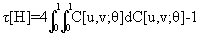Note that for any copula, the parameter θ corresponding to a desired τ[H] may be obtained by solving the above equation as a function of θ.

For more details on copulas, see the monographs by Nelsen  and Joe  and for a review article with biostatistical applications see Owzar and Sen .

Notations and assumptions

The actual time-to-event variables are denoted by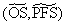respectively. Furthermore, the joint distribution ofis generated by a known parametric copula C[u,v;θ]. The marginal distribution function of OS and PFS are assumed to be exponential with rates λOS and λPFS respectively. The administrative censoring distribution is uniform on the interval [FU,FU+AP], where AP denotes the accrual period and FU denotes the follow-up time. Draw Z from a uniform distribution on [FU,FU+AP]. What is observed is (OS, ΔOS, PFS, ΔPFS), where observed time-to-event variable is then defined as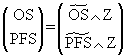where x ∧ y denote the minimum of x and y, and the event indicators are defined as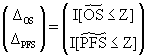Description of simulations

We conducted extensive simulations to evaluate the impact of alpha allocation on the overall type I error rate, marginal type I error rates for each endpoint and the proportion of time that we do not terminate the trial early using the SPARC trial as the motivating example. Because the majority of patients are expected to experience disease progression before dying, PFS in essence is the same as another time-to-event endpoint known as time to progression (TTP). Time to progression is defined as the interval between time of randomization to time of disease progression.

The SPARC trial was a phase III trial where 912 men with CRPC were to be randomized with 2:1 allocation ratio to either an experimental arm or a placebo. The trial duration was 44 months, with 26-months and 18 months for the accrual and follow-up period, respectively. The median PFS and OS times in the placebo arm were assumed to be 3 months and 12-months, similar to the SPARC trial. The marginal distributions of OS and PFS were generated with exponential distributions with hazard rates of 0.231 and 0.058. The censoring times were drawn from a uniform distribution on [18, 18+26] as described in section 2.1. The failure times and censoring times were generated completely independently.

We considered different simulation conditions by varying the following: allocation of type I error rate (equal vs. unequal), number of interim analysis for the PFS and OS endpoints (one or two or three), degree of dependence, as measured by Kendall's τ, between the two endpoints (τ = 0, 0.10, 0.30, 0.50, 0.70) and family of copula (normal, Frank, Gumbel) using a two-stage procedure. In the first stage, the Bonferroni correction was used where the global type I error rate was allocated to each of the endpoints. Two scenarios were considered: the type I error rate was equally split between the two endpoints (i.e=0.025) or unequal type I error rate was assumed for the PFS and the OS endpoints: (0.03 for PFS and 0.02 for OS, 0.04 for the OS and 0.01 for the PFS). In the next stage, once the alpha level for each endpoint was decided, we used the O'Brien-Fleming approach to design the boundary for the interim and final analysis for each endpoint.

For each of the above scenarios, 10,000 simulated datasets were generated. We were interested in testing the null hypothesis for the PFS endpoint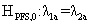(6)

against the alternative hypothesis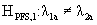(7)

where λ1aand λ2a are the hazard rates of progression in groups 1 and 2, respectively. In addition, we were interested in testing the null hypothesis for the OS endpoint: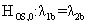(8)

against the alternative hypothesis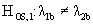(9)

where λ1band λ2b are the hazard rates of death in groups 1 and 2.

The empirical global type I error rate was estimated as the proportion of simulated datasets that would reject the null hypothesis of no difference in PFS or no difference in OS or both. The associated hypotheses are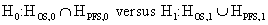(10)

Furthermore, the empirical type I error rate for the PFS endpoint was estimated as the proportion of simulated datasets that would reject the null hypothesis of no difference in PFS (equation 6). Similarly, the empirical type I error rate for the OS endpoint was estimated as the proportion of simulated datasets that would reject the null hypothesis of no difference in OS (equation 8). Under the alternative hypothesis, we were interested in evaluating the global power, marginal power for each endpoint and the proportion of exiting the trial early. In addition, the global power was estimated as the proportion of simulated datasets that would reject the null hypothesis of a difference in PFS (equation 7) or a difference in OS (equation 9) or both. The empirical power for the PFS endpoint (or the OS) was estimated as the proportion of simulated datasets that would reject the null hypothesis under the alternative hypothesis of a difference in the PFS or the OS or both. A copy of the code is provided online at this link: http://www.duke.edu/shalabi/JBB/simulate-code.R.

#### Results

Table 1 presents the empirical global type I error rate, empirical type I error rates for each endpoint and the proportion of not exiting the trial early at each look, assuming an equal allocation of the type I error rate = 0.025 for each endpoint. In addition, two analyses are assumed for the PFS and OS endpoints with O'Brien-Fleming type I error rate boundaries of 0.00146 and 0.02441. When τ = 0, the two endpoints are considered to be independent and the empirical type I error rate was 0.05. The empirical type I error rates for each of the endpoints were approximately 0.025. When normal copula and τ = 0.10 was assumed, the global type I error rate was 0.05. The empirical type I error rates were 0.0243 for the PFS endpoint and 0.0265 for the OS endpoint. Similar patterns were observed when τ = 0.30 and τ = 0.50. The empirical global type I error rate, however, decreased when τ = 0.70. The empirical global type I error rate is 0.039, with error rates of 0.0229 and 0.0259, for the PFS and OS endpoints, respectively. Again, similar patterns were observed when Frank and Gumbel copulas were utilized. The stronger the dependence between the PFS and OS endpoints, the smaller were the empirical global type I error rates. On the other hand, the empirical type I error rates for the PFS and OS endpoints were very close to the nominal values of 0.025.

Type of Copula      τ Global Type I                           PFS                        OS
Error Rate 1st Interim Final Analysis Type I Error 1st Interim Final Analysis Type I Error
Type-I error rate   0.05 0.00146 0.02441 0.025 0.00146 0.02441 0.025
Independence 0 0.051 0.0015 0.0244 0.0259 0.0015 0.0251 0.0257
Normal 0.10 0.050 0.0017 0.0226 0.0243 0.0023 0.0254 0.0265
0.30 0.047 0.0013 0.0219 0.0232 0.0022 0.0259 0.0267
0.50 0.047 0.0021 0.0251 0.0273 0.0017 0.0255 0.0264
0.70 0.039 0.0005 0.0224 0.0229 0.0019 0.0259 0.0265
Frank 0.10 0.051 0.0017 0.0254 0.0271 0.0012 0.0242 0.0247
0.30 0.044 0.0014 0.0228 0.0242 0.0012 0.0217 0.0221
0.50 0.046 0.0016 0.0244 0.0260 0.0014 0.0247 0.0253
0.70 0.040 0.0020 0.0234 0.0254 0.0017 0.0217 0.0226
Gumbel 0.10 0.046 0.0014 0.0210 0.0224 0.0012 0.0246 0.0248
0.30 0.051 0.0016 0.0230 0.0246 0.0017 0.0283 0.0286
0.50 0.041 0.0014 0.0220 0.0234 0.0016 0.0220 0.0227
0.70 0.042 0.0015 0.0257 0.0257 0.0018 0.0246 0.0253

Table 1: Empirical global type I error rates, empirical significance level for each endpoint and proportion of not terminating the trial early assuming equal allocation of type I error rate of 0.025 for each endpoint and using O'Brien-Fleming boundaries; results based on 10,000 simulations.

When τ = 0, the average proportion of not terminating the trial early at the first look was 0.0015 for both endpoints. The proportion of not terminating early at the final analysis were 0.0244 and 0.0251 for the PFS and OS endpoints. Overall, these values were very close to the nominal values for the first interim and final analyses with a few exceptions. When τ = 0.70, the proportion of exiting the trial early were 0.0005 and 0.0020 when normal and Frank copula's were assumed.

Table 2 lists the empirical global power, empirical power for each of two endpoints and the proportion of terminating the trial early based on 10,000 simulations. The overall power was above 0.95 and the empirical powers were 0.852 and 0.856 for the PFS and OS endpoints, respectively. Overall, the power was about 0.85 for each of the two endpoints, regardless of the degree of dependence (i.e. value of τ) and type of copula that were assumed.

Type of Copula      τ Global Power                            PFS                             OS
1st Interim Final Analysis Power 1st Interim Final Analysis Power
Independence 0 0.979 0.206 0.647 0.853 0.221 0.855 0.856
Normal 0.10 0.969 0.205 0.645 0.851 0.211 0.849 0.849
0.30 0.955 0.202 0.646 0.848 0.217 0.852 0.853
0.50 0.934 0.209 0.639 0.849 0.216 0.860 0.860
0.70 0.912 0.203 0.644 0.847 0.209 0.855 0.856
Frank 0.10 0.974 0.203 0.646 0.850 0.206 0.856 0.857
0.30 0.957 0.207 0.645 0.852 0.215 0.856 0.857
0.50 0.938 0.203 0.647 0.850 0.218 0.852 0.853
0.70 0.912 0.204 0.640 0.844 0.215 0.854 0.855
Gumbel 0.10 0.969 0.209 0.638 0.846 0.221 0.853 0.854
0.30 0.947 0.208 0.638 0.846 0.217 0.854 0.854
0.50 0.929 0.208 0.639 0.847 0.210 0.857 0.857
0.70 0.909 0.212 0.638 0.850 0.216 0.854 0.855

Table 2: Empirical global power, power for each endpoint and proportion of terminating the trial early assuming equal allocation of type I error rate of 0.025 for each endpoint and using O'Brien-Fleming boundaries; results based on 10,000 simulations.

In Table 3, we present the empirical global type I error rate, marginal type I error rates for each endpoint and the proportion of not exiting the trial early at each look assuming equal allocation of the type I error rate of 0.025 for each endpoint. We assumed two analyses for the PFS and OS endpoints each at 50% and 100% of the total information, but the Pampallona and Tsiatis type I error rate boundaries of 0.00067 and 0.02479 were used. We observed similar trends as we did in Table 1.

Type of Copula      τ Global Type I                         PFS                         OS
Error Rate 1st Interim Final Analysis Type I Error 1st Interim Final Analysis Type I Error
Type-I error rate   0.05 0.00067 0.02479 0.025 0.00067 0.02479 0.025
Independence 0 0.054 0.0008 0.0280 0.0288 0.0008 0.026 0.0260
Normal 0.10 0.050 0.0007 0.0249 0.0256 0.0010 0.0251 0.0255
0.30 0.046 0.0008 0.0224 0.0232 0.0006 0.0256 0.0256
0.50 0.048 0.0010 0.0260 0.0270 0.0009 0.0259 0.0270
0.70 0.043 0.0005 0.0265 0.027 0.0006 0.0250 0.0253
Frank 0.10 0.049 0.0009 0.0224 0.0233 0.0006 0.0258 0.0262
0.30 0.049 0.0009 0.0242 0.0251 0.0007 0.0245 0.0253
0.50 0.046 0.0006 0.0265 0.0271 0.0009 0.0233 0.0234
0.70 0.044 0.0009 0.0250 0.0259 0.0005 0.0279 0.0279
Gumbel 0.10 0.051 0.0006 0.0262 0.0268 0.0002 0.0256 0.0257
0.30 0.049 0.0012 0.0250 0.0262 0.0008 0.0254 0.0255
0.50 0.042 0.0006 0.0234 0.0240 0.0005 0.0242 0.0243
0.70 0.041 0.0005 0.0254 0.0259 0.0004 0.0253 0.0254

Table 3: Empirical global type I error rates, empirical signficance level for each endpoint and proportion of not terminating the trial early assuming equal allocation of type I error rate of 0.025 for each endpoint and using Pampallona-Tsiatis boundaries; results based on 10,000 simulations.

Table 4 presents the empirical power for the global power, empirical power for each of two endpoints and the proportion of exiting the trial early assuming two analyses for the PFS and OS endpoints each at 50% and 100% of the total information with Pampallona and Tsiatis type I error rate boundaries of 0.00067 and 0.02479. Overall, the empirical powers for the PFS and OS endpoints were approximately 0.85 and the empirical global power was higher than 0.95 when τ was less than 0.7.

Typeof Copula      τ GlobalPower                          PFS                           OS
1stInterim Final Analysis Power 1stInterim Final Analysis Power
Independence 0 0.979 0.156 0.696 0.852 0.172 0.859 0.859
Normal 0.10 0.973 0.152 0.701 0.853 0.155 0.857 0.857
0.30 0.956 0.154 0.694 0.848 0.158 0.855 0.856
0.50 0.939 0.151 0.707 0.858 0.158 0.861 0.861
0.70 0.914 0.151 0.703 0.855 0.156 0.856 0.856
Frank 0.10 0.970 0.156 0.689 0.845 0.161 0.853 0.854
0.30 0.953 0.154 0.693 0.847 0.161 0.854 0.854
0.50 0.938 0.147 0.703 0.850 0.157 0.851 0.851
0.70 0.913 0.146 0.703 0.849 0.159 0.852 0.853
Gumbel 0.10 0.968 0.156 0.696 0.851 0.158 0.852 0.853
0.30 0.952 0.153 0.696 0.849 0.157 0.860 0.861
0.50 0.930 0.150 0.700 0.850 0.151 0.858 0.858
0.70 0.914 0.154 0.670 0.853 0.163 0.860 0.861

Table 4: Empirical global Power and power for each endpoint and proportion of terminating the trial early assuming equal allocation of type I error rate of 0.025 for each endpoint and using Pampallona-Tsiatis boundaries; results based on 10,000 simulations.

We also evaluated the empirical global type I error rate and type I error rates for each endpoint assuming unequal allocation of the error rate (Table 5). We allocated type I error rates of 0.03 and 0.02 for the PFS and OS endpoints, respectively. Using the O'Brien-Fleming approach, the type I error rate boundaries were 0.00042 and 0.02990 for the first and final analysis for PFS, whereas they were 0.0014 and 0.01938 for the OS endpoint. The empirical global type I error rate was 0.05, although it decreased to below 0.05 when was 0.5 or higher. The empirical marginal type I error rates for each endpoint were approximately 0.03 and 0.02.

Type of Copula      τ Global Type I                           PFS                             OS
Error Rate 1st Interim Final Analysis Type I Error 1st Interim Final Analysis Type I Error
Type-I error rate   0.05 0.00042 0.02990 0.03 0.0014 0.01938 0.020
Independence 0 0.048 0.0004 0.0305 0.0307 0.0009 0.0176 0.0178
Normal 0.10 0.051 0.0008 0.0310 0.0318 0.0011 0.0199 0.0202
0.30 0.049 0.0005 0.0294 0.0295 0.0016 0.0211 0.0220
0.50 0.046 0.0003 0.0312 0.0315 0.0006 0.0194 0.0195
0.70 0.039 0.0002 0.0302 0.0304 0.0001 0.0188 0.0192
Frank 0.10 0.045 0.0004 0.0254 0.0258 0.0013 0.0192 0.0196
0.30 0.049 0.0006 0.0300 0.0306 0.0015 0.0188 0.0194
0.50 0.045 0.0002 0.0302 0.0304 0.0016 0.0184 0.0191
0.70 0.041 0.0001 0.0301 0.0302 0.0013 0.0176 0.0183
Gumbel 0.10 0.050 0.0005 0.0296 0.0301 0.0016 0.0192 0.0200
0.30 0.047 0.0017 0.0317 0.0324 0.0013 0.0170 0.0173
0.50 0.043 0.0005 0.0299 0.0304 0.0009 0.0178 0.0181
0.70 0.042 0.0005 0.0316 0.0321 0.0011 0.0184 0.0190

Table 5: Empirical global type I error rates, empirical significance level for each endpoint and proportion of not terminating the trial early using O'Brien-Fleming boundaries and assuming unequal allocation of the type I error rate of 0.03 for PFS and 0.02 for OS; results based on 10,000 simulations.

In Table 5, we observed that the empirical global power was greater than 0.95 when τ was small to moderate (i.e. less than 0.7). The global power decreased to 0.91 when τ = 0.7. Overall, the empirical powers were approximately 0.86 and 0.84 for the PFS and the OS endpoints, respectively. In addition, we considered an unequal allocation of the type I error rate where a type I error rate of 0.01 was used for PFS and 0.04 for the OS. We observed similar patterns with empirical global type I rates of 0.05 and type I error rates of 0.01 and 0.04 for the PFS and OS endpoints, respectively (Table not presented). We also considered three analyses for the PFS endpoint at 50%, 75% and 100% events and two analyses for the OS endpoint. The empirical type I error rates, marginal type I error rates, and powers were similar to we observed for the two analysis scenario.

Application

The SPARC trial was an international double-blinded phase III trial where 950 men with CRPC were randomized with 2:1 allocation ratio to satraplatin (experimental arm) or a placebo . The trial was designed so that the PFS endpoint has a 85% power to detect a hazard ratio (HR) of 1.3. Under the alternative hypothesis of a difference in PFS, 700 PFS events were expected to occur at about 24 months. Similarly, under the alternative hypothesis of the OS endpoint, the study was designed with 85% power to detect a HR=1.3. The 700 deaths were projected to occur at 44 months after trial activation.

The Bonferroni correction was used in which the type I error rate of 0.05 was equally split between the two endpoints. In addition, two analyses were to be performed on the PFS endpoint: at 50% PFS events and at 100% of the total events which were projected to occur at 15 and 24 months after study activation. Similarly, for the OS endpoint, one interim analysis was to be performed at 50% and the final analysis when 700 deaths have been observed. A two-stage procedure was used to adjust for the type I error rate. First, the Bonferroni method was used to adjust for the type I error rate between the two endpoints. Once the type I error rate was allocated, we used the O'Brien-Fleming method to derive the z-score boundaries and the type I error rates for each of the interim and final analysis so the overall global type I error rate is preserved at the pre-specified type I level. The trial was monitored by the data monitoring committee for both the PFS and the OS endpoints.

#### Discussion

The present study empirically assessed the global type I error rate and the marginal type I error rates for two endpoints when the alpha level was allocated between two dependent time to-event endpoints. The results of the simulations demonstrate that we control for the global type I error and type I rates for both of the endpoints under the null hypothesis. When τ, which is a measure of the dependence between the two endpoints, was close to zero, the type I error rates were very close to the Bonferroni corrected alpha level. Not surprisingly, the empirical global type I error rate and type I error rates for each endpoint were smaller than the nominal values as the value of τ increased.

In addition, the global power and individual power for each endpoint were attained at the desired level under the alternative hypotheses. As expected, the power was very close to the desired power when τ was equal to or close to zero. As the value of τ increased, the empirical power was approximately 0.90, but the powers for each endpoint were controlled at the desired levels of 0.85 (Table 6).

Typeof Copula       τ GlobalPower                             PFS                             OS
Analysis   1stInterim Final Analysis Power 1stInterim Final Analysis Power
Independence 0 0.978 0.121 0.745 0.866 0.193 0.832 0.834
Normal 0.10 0.971 0.124 0.748 0.872 0.216 0.832 0.834
0.30 0.952 0.129 0.736 0.865 0.204 0.826 0.827
0.50 0.932 0.132 0.732 0.864 0.210 0.836 0.837
0.70 0.918 0.120 0.748 0.868 0.206 0.840 0.841
Frank 0.10 0.970 0.123 0.740 0.863 0.209 0.833 0.834
0.30 0.952 0.121 0.739 0.860 0.211 0.834 0.831
0.50 0.936 0.121 0.749 0.869 0.205 0.831 0.831
0.70 0.917 0.130 0.739 0.869 0.217 0.835 0.836
Gumbel 0.10 0.970 0.125 0.744 0.869 0.216 0.832 0.833
0.30 0.950 0.124 0.749 0.873 0.204 0.832 0.833
0.50 0.930 0.122 0.745 0.866 0.208 0.837 0.838
0.70 0.908 0.122 0.748 0.870 0.207 0.829 0.831

Table 6: Empirical global power, power for each endpoint and proportion of terminating the trial early using O'Brien-Fleming boundaries and assuming unequal allocation of the type I error rate of 0.03 for PFS and 0.02 for OS; results based on 10,000 simulations.

Our simulations assumed two interim analyses at 50% and 100% of the information for the PFS and the OS endpoints. In addition, we considered different combinations with three interim analyses at 50%, 75% and 100% for PFS and two interim analyses for OS. These scenarios were chosen based on common practices in industry sponsored phase III trials in oncology. Nevertheless, depending on the specifics of a trial, this approach will allow for the inclusion of more interim looks. In addition, varying strategies may be used to best allocate the type I error rate. For instance, if a drug is promising, a statistician may allocate less of the type I error rate on the intermediate endpoint, but reserve a large proportion of type I error rate on the primary endpoint that will be used in the full approval of the drug by the relevant regulatory authorities.

Another consideration is how to estimate the dependence between the two endpoints. In our example, we expected the two endpoints of PFS and OS to be highly dependent. We estimated τ based on historical data that were collected as part of phase II and phase III trials in men with CRPC. The estimated τ ranged from 0.31-0.50 depending on the definition of the PFS endpoint .

Although we have used PFS as the intermediate endpoint, in our example PFS and TTP were similar endpoints as the majority of the patients (96%) were anticipated to experience progression before death . In some cancers, however, patients may die before evidence of progression. And as such, more elaborate simulations to address this issue could be implemented.

The results of our simulations were robust regardless of the copula that we assumed. We are not able to recommend a choice of copula, but a graphical method discussed in Wang and Wells may help the reader in this respect . One advantage of using parametric copula is that they are flexible tools and can describe the dependence between two endpoints by a single parameter. The bivariate normal model is a special case of our model, that is, a Gaussian copula with normal marginals. They are computationally easy to implement and another advantage is that most of the literature on sequential methods is based on normal distribution.

In summary, the univariate monitoring approach seems to work if the dependence between the two endpoints is not too large. This approach is intuitive and easy to implement. Most available software can compute the sequential boundaries. The main drawback of using the Bonferroni correction is that it may be conservative.

#### Acknowledgements

This research was supported in part by National Institutes of Health Grant CA 155296-1A1. We thank Dr. Kouros Owzar for his valuable comments and his help in programming in R. We also thank the reviewers for their comments.

#### References

Select your language of interest to view the total content in your interested language

### Article Usage

• Total views: 12050
• [From(publication date):
specialissue-2013 - Dec 14, 2019]
• Breakdown by view type
• HTML page views : 8248Can't read the image? click here to refresh